MORE IN Network Analysis
Total marks: --
Total time: --
INSTRUCTIONS
(1) Assume appropriate data and state your reasons
(2) Marks are given to the right of every question
(3) Draw neat diagrams wherever necessary

1 (a) Find the equivalent resistance between the terminal A and B in the network shown in Fig Q1 (a) using Star-Delta transformation.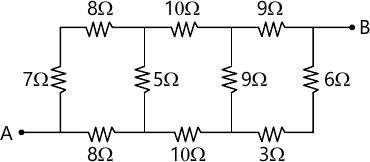6 M
1 (b) Find the power delivered by the dependent voltage source in the circuit shown in Fig Q1(b) by mesh current method.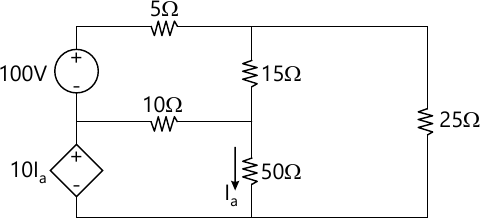6 M
1 (c) Find the current i, in the circuit shown in Fig Q1 (c) using Nodal Analysis.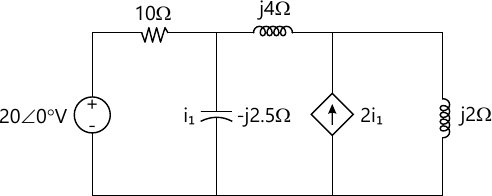8 M

2 (a) Define the terms tree, cotree, link, cutset schedule and Tie set schedule.
10 M
2 (b) Draw the graph of the network shown in Fig. Q2(b). Write the cut set schedule and find all node voltages, branch, voltages and branch currents. Assume branches (2) and (3) ro form the tree.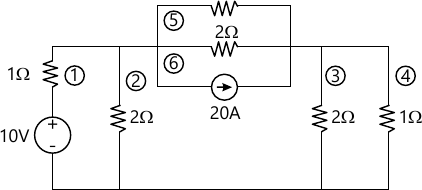10 M

3 (a) Find Ix for the circuit shown in figure Q3(a) using the principle of superposition.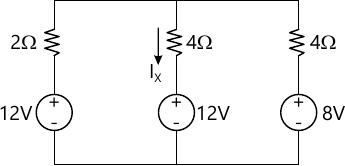6 M
3 (b) State and explain Millman's theorem.
6 M
3 (c) Verify reciprocity theorem for the circuit shown in Fig. Q3(c) with response I.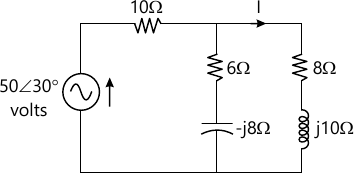8 M

4 (a) State and explain the Vinin's theorem.
6 M
4 (b) In the circuit shown in Fig Q4(b), find the value of the current through the 667Ω resistor using Norton's theorem.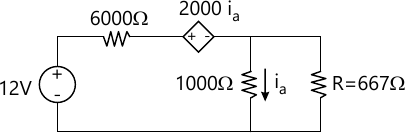6 M
4 (c) In the circuit shown in Fig Q4(c), find the value of RL for which maximum power is delivered. Also find the maximum power that is delivered to the load RL.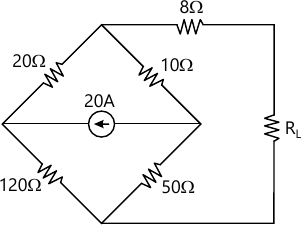8 M

5 (a) It is required that a series RLC circuit should resonate at 500KHz. Determine the values of R, L and C if the Bandwidth of the circuit is 10 Khz and its impedance is 100Ω at resonance. Also find the voltages across L and C resonance if the applied voltage is 75 volts.
10 M
5 (b) Derive an expression for the resonant frequency of a parallel resonant circuit. Also shown that the circuit is resonant at all frequencies if $$R_L = R_C =\sqrt{ \dfrac {L}{C} }$$ where RL = Resistance in the indicator branch, RC = Resistance in the capacitor branch.
10 M

6 (a) In the circuit shown in Fig Q6(a), the switch K is changed from position A to B at t=0, steady state having been leached before switching. Calculate $$i, \ \dfrac {di}{dt}\ \text{ and } \dfrac {d^2i}{dt^2} \text { at }t=0^+$$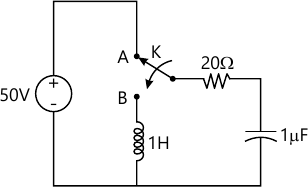10 M
6 (b) In the Network shown in Fig Q6(b), steady state is leached with switch-K open. The switch is closed at time t=0. Solve for $$i_1, \ i_2, \ \dfrac {di_1}{dt} \text { and } \dfrac {di_2}{dt} \text { at }t=0^+.$$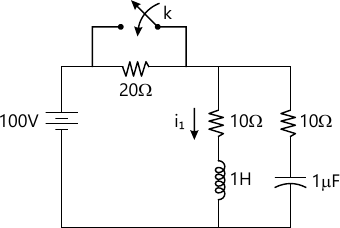10 M

7 (a) Obtain the Laplace transform of the Periodic signal shown if Fig. Q7(a)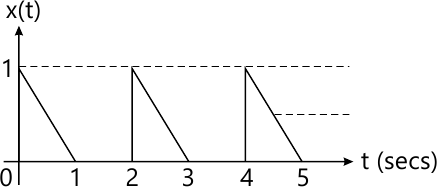10 M
7 (b) Find the convolution of h(t)=e-1 and f(t)=e-2t.
4 M
7 (c) State and prove the initial value theorem.
6 M

8 (a) Derive y-parameters and Transmission parameters of a circuit in terms of its z-parameters.
10 M
8 (b) Find the z parameters and h-parameters for the circuit shown in Fig. Q8(b)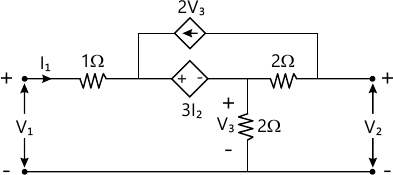10 M

More question papers from Network Analysis